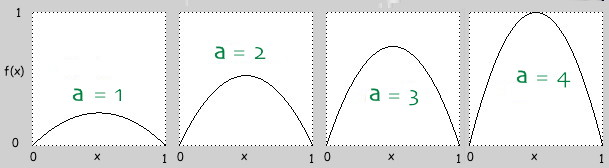◀ Navigate through the Chaos pages ►

# The Logistic Map 1 — Single Steps

What happens to the sequence x, f(x), f(f(x)), etc... for the particular function a∙x∙(1-x)?

## Brief Explanation

If you are unfamiliar with the terminology of iterated functions and orbits, you should visit the preceding page in this series, by clicking the “Previous” link at the top of this page.

First, what does a∙x∙(1-x) look like? Here are some graphs of the function, with the coefficient a ranging from 1 to 4.You can see that both x and f(x) always stay between 0 and 1. (Including those boundary values.) So you should always set the value of a between 0 and 4, and the starting value of x between 0 and 1.

To set the value of a, you can use the slider, you can use the buttons below, or you can just type in a value yourself. Next, type a starting value for x, between 0 and 1. Then click the “Step” button.

## Try It Yourself

f(x) = a∙x∙(1-x)Orbit values x, f(x), f(f(x)), ...
∙x∙(1-x) =
This sets x to be f(x)

0 4
Slider for setting a

### Interesting values for a

• a = This has a single attractor at value 0. This should be no surprise!
• In fact, all values of a from 0 to 1 have orbits that head to zero.
• a = This has a single attractor at value 0.5.
• At a = 3 the orbit turns into a period-2 cycle. The next three values show the period-doubling behavior as a increases.
• a = The orbit values settle down to a period-2 cycle.
• a = The values fall into a period-4 cycle.
• a = The values fall into a period-8 cycle. (Takes a while to settle in.)
• From here up to the value a ≈ 3.57 the periods keep doubling, and every power of 2 occurs. After that, things are rather complicated until a reaches 1 + 2√2 ≈ 3.828, at which point a cycle of length 3 appears.
• a = The values fall into a period-3 cycle!
• After this, as a increases the periods again double, to 6, 12, 24, etc. until chaos breaks out again.
• a = The values change chaotically.

## Remarks

It took the modern age of calculators and computers for this kind of investigation to really get moving, although earlier mathematicians did catch glimpses of these sorts of patterns. (To answer a challenge from the previous page, to find an attractor of period 5, try a = 3.74.) The next page will give you a more pictorial view of how this particular function looks when graphed and iterated.

◀ Navigate through the Chaos pages ►